# Materialized views with Practical approach in Cassandra

• Last Updated : 27 Nov, 2019

In this article, we will discuss a practical approach in Cassandra. In Cassandra, the Materialized view handles the server-side de-normalization and in between the base table and materialized view table ensure the eventual consistency. let’s understand with an example.

Let’s first define the base table such that student_marks is the base table for getting the highest marks in class. In this base table subject_name, student_name and marks are the columns. We will create a materialized view table of the base table and queried the following query given below.

From materialized view find the highest marks of a student in a class.

Example: Base Table – student_marks

```CREATE TABLE student_marks
(
subject_name text,
student_name text,
marks int,
PRIMARY KEY (subject_name, marks)
); ```

Output: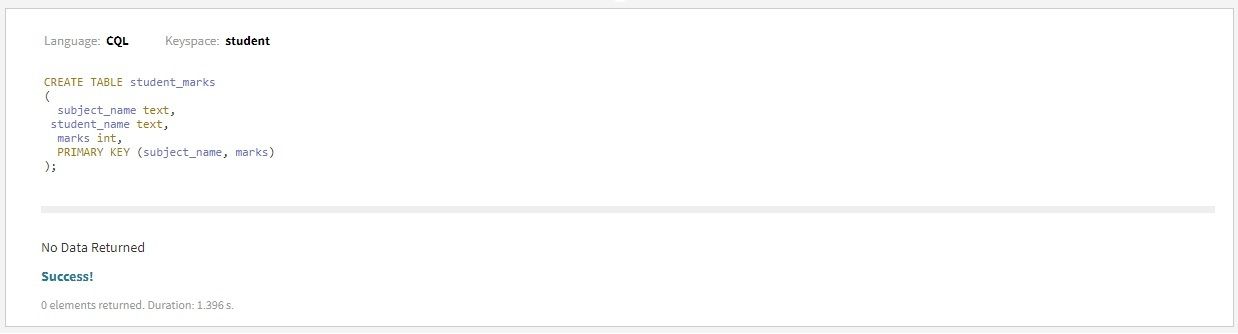Now, let’s discuss the materialized view of the above base table in which we will create the view which presents the highest marks of a student in a class.

To create the materialized view (materialized view table – everytime_high_marks) we provide a simple select statement and the primary key to use for this view. Specifying the CLUSTERING ORDER BY allows us to reverse sort the highest marks so we can get the highest marks by simply selecting the first item in the partition.

To create a materialized view used the following CQL query.

```CREATE MATERIALIZED VIEW everytime_high_marks AS
SELECT student_name, marks, subject_name
FROM student_marks
WHERE student_name IS NOT NULL
AND marks IS NOT NULL
AND subject_name IS NOT NULL
PRIMARY KEY (subject_name, marks)
WITH CLUSTERING ORDER BY (marks desc);  ```

Output: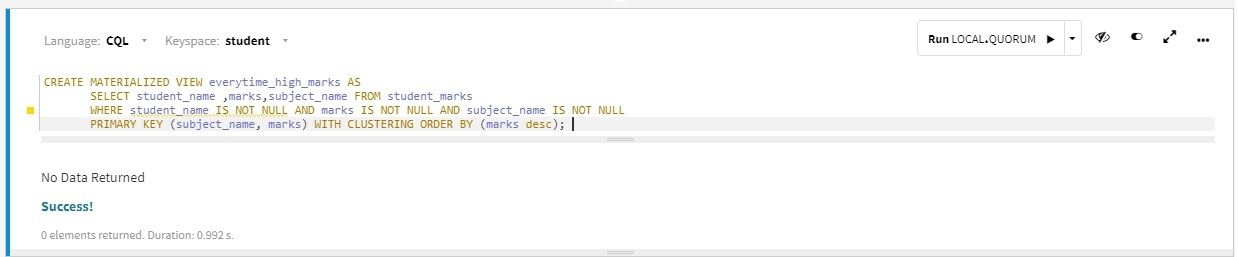Now, first we insert data into the base table and it will reflect materialized view as well. After inserting data we can query from the materialized view table and get the desired result for the CQL query. for practical, we are using DataStax Studio to run all the queries to verify the CQL query.

To insert data into the base table used the following CQL query.

```INSERT INTO student_marks (student_name, subject_name, marks)
VALUES ('Ashish', 'Cassandra DB', 95);
INSERT INTO student_marks (student_name, subject_name, marks)
VALUES ('Rana', ' Cassandra DB ', 80);
INSERT INTO student_marks (student_name, subject_name, marks)
VALUES ('Amit', ' Cassandra DB ', 85);
INSERT INTO student_marks (student_name, subject_name, marks)
VALUES ('Shivang', ' Cassandra DB ',90);
INSERT INTO student_marks (student_name, subject_name, marks)
VALUES ('Kartikey', ' Cassandra DB ',92);
INSERT INTO student_marks (student_name, subject_name, marks)
VALUES ('Dhruv', 'Cassandra DB',93);
INSERT INTO student_marks (student_name, subject_name, marks)
VALUES ('Rahul', 'Cassandra DB',91);
INSERT INTO student_marks (student_name, subject_name, marks)
VALUES ('Gaurav', 'Cassandra DB',96); ```

Output: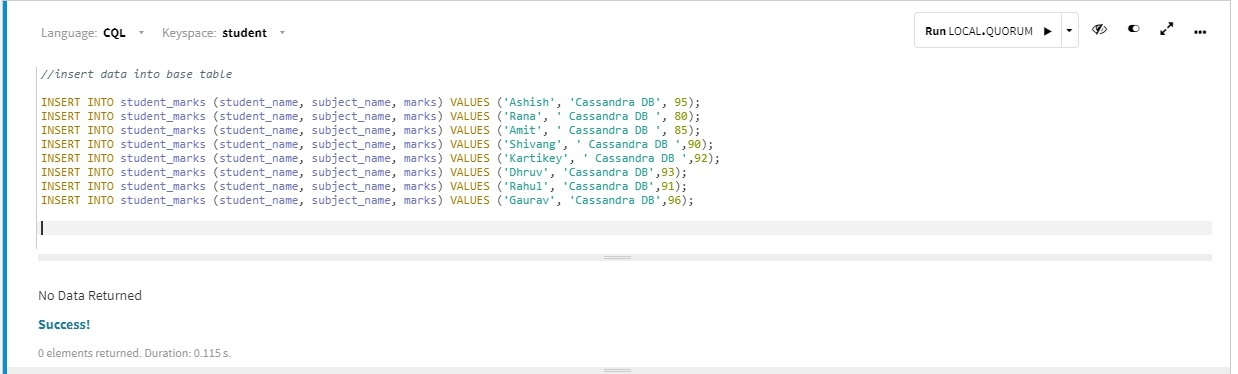Figure – Insert data into base table

```SELECT *
FROM everytime_high_marks
WHERE subject_name = 'Cassandra DB'; ```

Output: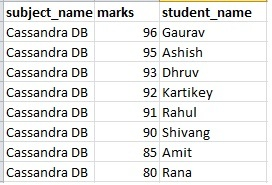Figure – MV Output

Now, we can search for a student who has marks the highest ever in a class for a given subject. we can use the materialized view table to get the result that we are expecting. Let’s have a look.

```SELECT student_name, marks
FROM everytime_high_marks
WHERE subject = 'Cassandra DB' limit 1; ```

Here, we can use the ‘subject’ column with WHERE clause because it is the primary key to materialized view too.

Output: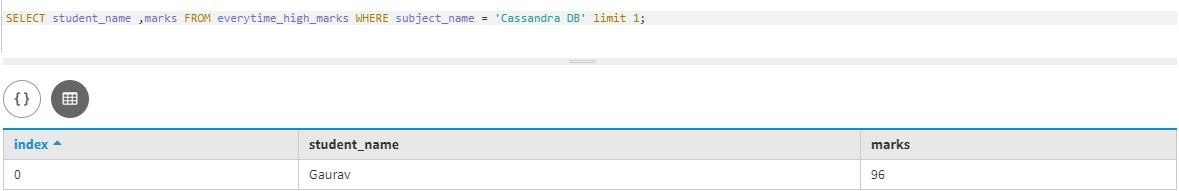As shown in the output table Gaurav has 96 marks which are the highest in class.

My Personal Notes arrow_drop_up